# Rényi test

Jump to: navigation, search

A statistical test used for testing a simple non-parametric hypothesis(cf. Non-parametric methods in statistics), according to which independent identically-distributed random variableshave a given continuous distribution function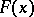, against the alternatives: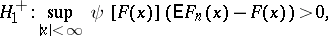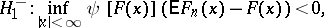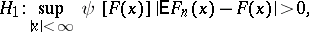where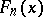is the empirical distribution function constructed with respect to the sampleand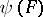,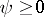, is a weight function. Ifwhereis any fixed number from the interval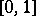, then the Rényi test, which was intended for testing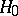against the alternatives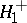,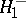,, is based on the Rényi statistics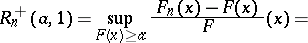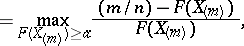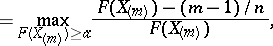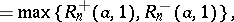where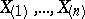are the members of the series of order statistics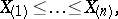constructed with respect to the observations.

The statistics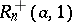and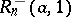satisfy the same probability law and, if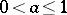, then(1)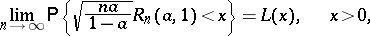(2)

where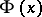is the distribution function of the standard normal law (cf. Normal distribution) andis the Rényi distribution function,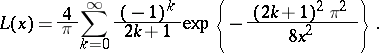If, then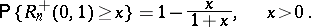It follows from (1) and (2) that for larger values ofthe following approximate values may be used to calculate the-percent critical values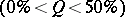for the statisticsand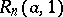: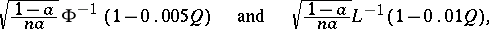respectively, whereand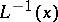are the inverse functions toand, respectively. This means that if, then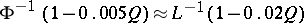.

Furthermore, if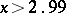, then it is advisable to use the approximate equation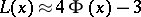when calculating the values of the Rényi distribution function; its degree of error does not exceed.

In addition to the Rényi test discused here, there are also similar tests, corresponding to the weight function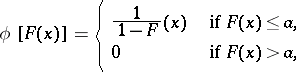whereis any fixed number from the interval.